﻿ SQL Server HAVING Clause with Examples

# SQL Server HAVING Clause with Examples

Unlike the WHERE clause, the HAVING clause in SQL Server is used when you have Aggregate functions (like SUM(), COUNT() etc.) in your SQL query. There are different ways you can use the HAVING clause in an SQL query.

image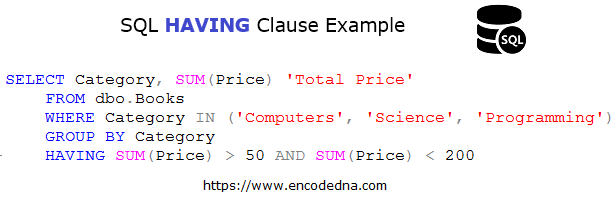## Syntax HAVING

```SELECT Col1, FUNCTION(Col2)
FROM dbo.table_name
GROUP BY Col1
HAVING CONDITION```

Let's see some examples.

I have a table named dbo.Books, which I’ll use in my examples here. Here’s the table. Create this table in your SQL Server database or you can use any other table that has some numeric values. Yes, the table must have number values, since you need to use Aggregate functions like SUM(), COUNT() etc.

image (table)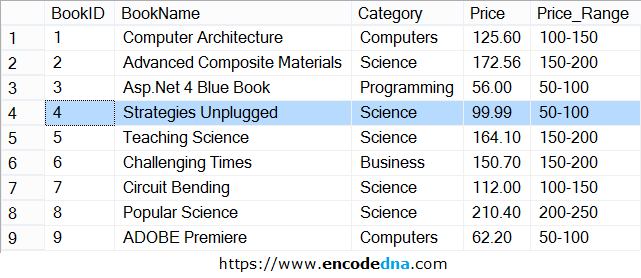## Using HAVING clause in SQL Server

See the above table again. There are different categories (like Computer, Science etc.) with a price tag. I can use the SUM() function (an aggregate function) to get the total price of each category. Like this,

```SELECT Category, SUM(Price) 'Total Price'
FROM dbo.Books
GROUP BY Category```

image (result)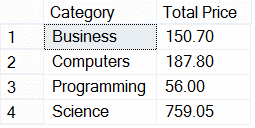It shows the sum of the price, grouped by the Category (or for each group).

Note: Since I am using a Column name (Category) with the aggregate SUM() functin in my query, I have to use the GROUP BY clause. Or else, it will throw an error.

Now, I want to filter it further. I want to get the total price for particular categories, whose total price is more than 150. So, if the sum of the price is more than 150, show it or else ignore it.

Here I need to use the HAVING clause.

```SELECT Category, SUM(Price) 'Total Price'
FROM dbo.Books
GROUP BY Category
HAVING SUM(Price) > 150```

image (result)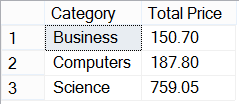Another HAVING clause example and this time I am using the COUNT() function.
Remember, you can use the HAVING clause with any aggregate function.

```SELECT COUNT(BookID) C, Category
FROM dbo.Books
GROUP BY Category
HAVING COUNT(Category) >= 2```

This above query returns categories, which have 2 or more rows in the table.

image (result)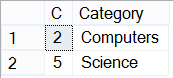## Using HAVING and WHERE clause together

This is another interesting and important query, which you’ll often use. Using the HAVING clause with the WHERE clause.

Although, both HAVING and WHERE are used to filter rows (using conditions) in a table, both have different usages. See this query.

```SELECT Category, SUM(Price) 'Total Price'
FROM dbo.Books
WHERE Category IN ('Computers', 'Science', 'Programming')
GROUP BY Category, Price
HAVING SUM(Price) > 150```

I am first filtering the Categories using the WHERE clause and later filtering it further using the HAVING clause.

## Using HAVING clause with Multiple values

With multiple values, I mean multiple conditions. For example, now I want to use a range of values with HAVING and filter rows based on that range.

```SELECT Category, SUM(Price) 'Total Price'
FROM dbo.Books
WHERE Category IN ('Computers', 'Science', 'Programming')
GROUP BY Category
HAVING SUM(Price) > 50 AND SUM(Price) < 200```

The range I have set is more than 50 and less than 200.

Talking about multiple values in HAVING, you can also do this.

```SELECT Category, SUM(Price) 'Total Price'
FROM dbo.Books
GROUP BY Category
HAVING Category LIKE '%comp%' and SUM(Price) < 200```

Hope this is useful. These are just few basic examples, however, it will you give a clear idea about using the HAVING clause in an SQL query.